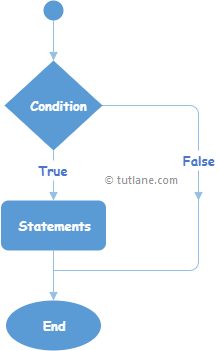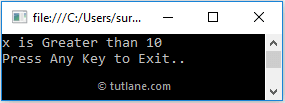# C# If Statement with Examples

In c#, if statement or condition is used to execute the block of code or statements when the defined condition is true.

Generally, the statement that will execute based on the condition is known as a “Conditional Statement” and the statement is more likely a block of code.

## Syntax of C# if Statement

Following is the syntax of defining if statement in c# programming language.

if (bool_expression) {
// Statements to Execute if condition is true
}

If you observe above if statement syntax, the statements inside of if condition will be executed only when the “bool_expression” returns true otherwise the statements inside of if condition will be ignored for execution.

Following is the simple example of using if statement in the c# programming language.

int x = 20;
if (x >= 10)
{
Console.WriteLine("Number Greater than 10");
}

If you observe the above example, the Console statement will be executed only when the defined condition (x >= 10) returns true.

## C# If Statement Flow Chart Diagram

The following is the flow chart diagram that will represent the process flow of if statement in c# programming language.If you observe the above c# if statement flow chart, if the defined condition is true, then the statements within the if condition will be executed; otherwise, the if condition will come to an end without executing the statements.

## C# If Statement Example

Following is the example of defining if statement in c# programming language to execute the block of code or statements based on a Boolean expression.

using System;

namespace Tutlane
{
class Program
{
static void Main(string[] args)
{
int x = 20, y = 10;
if (x >= 10)
{
Console.WriteLine("x is Greater than 10");
}
if (y <= 5)
{
Console.WriteLine("y is less than or equals to 5");
}
Console.WriteLine("Press Enter Key to Exit..");
}
}
}

If you observe the above code, we defined two if conditions to execute statements based on defined variables (x, y) values.

## Output of C# If Statement Example

When we execute the above c# program, we will get the result as shown below.If you observe the above result, only one if statement condition is true; that’s why only one statement is printed on the console window.

This is how we can use if statement in c# programming language to execute the block of code or statements based on our requirements.